# biophysics.ppt

31 de Mar de 2023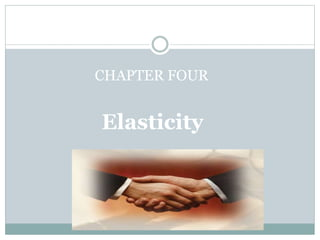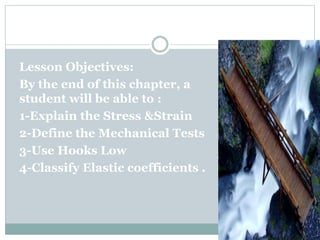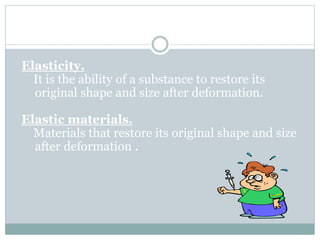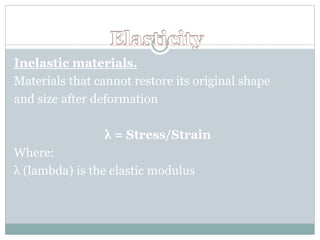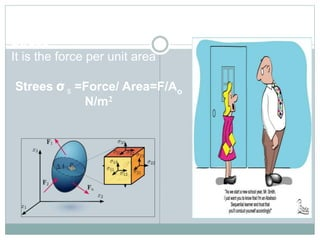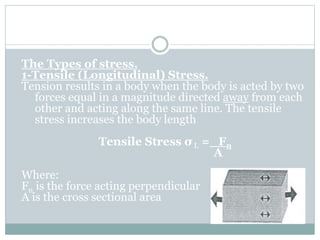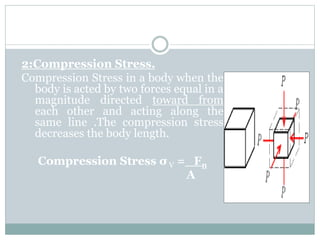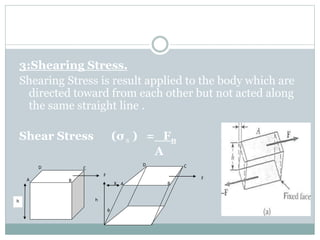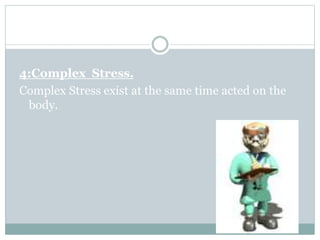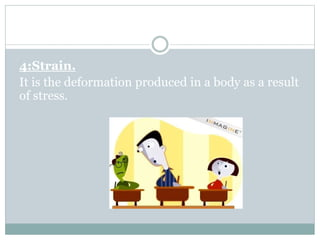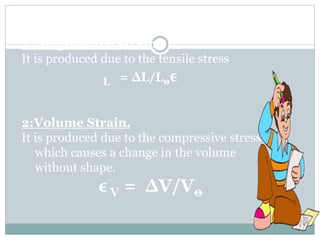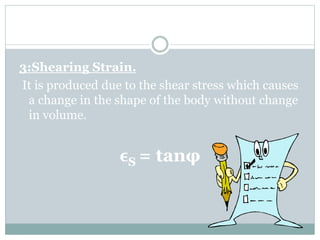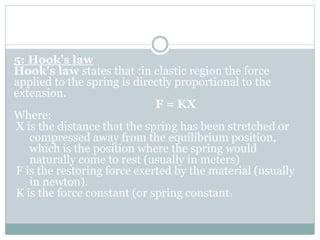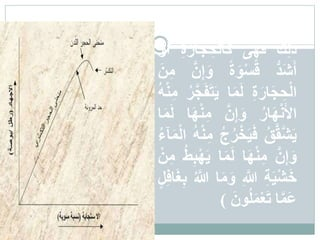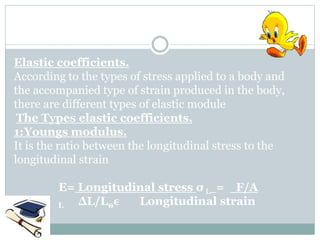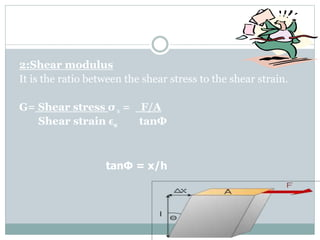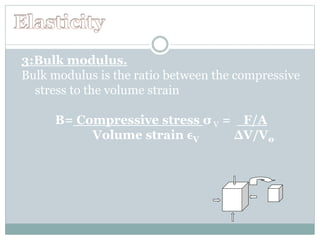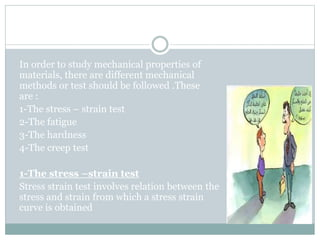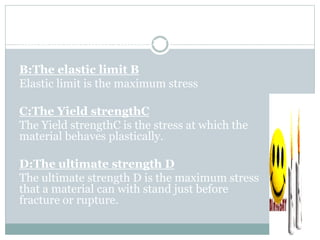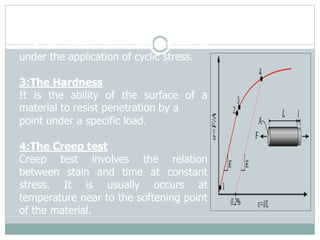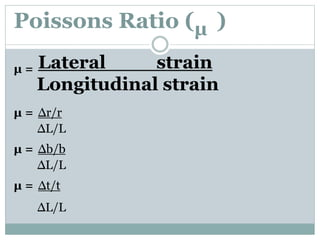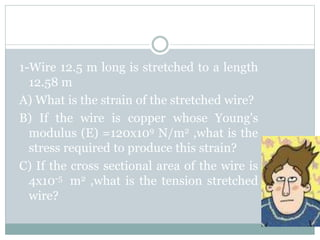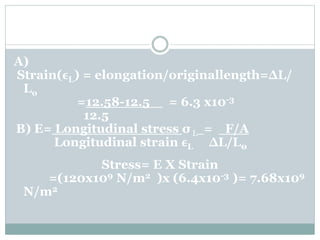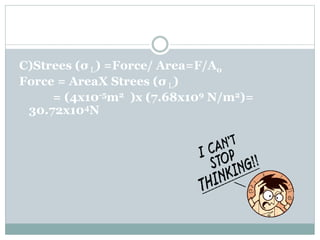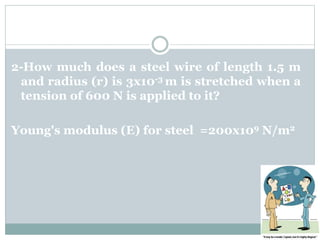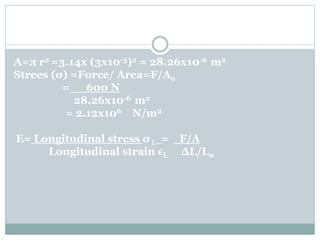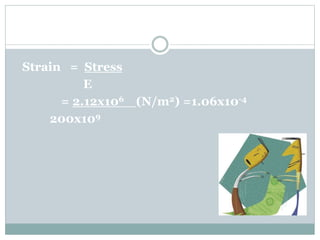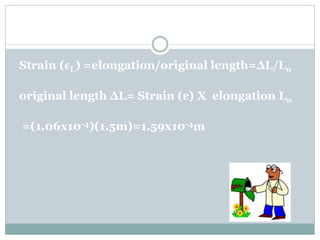1 de 31

### biophysics.ppt

1. ‫د‬ / ‫احمد‬ ‫مصطفى‬ ‫هالة‬ ‫الطبيعي‬ ‫العالج‬ ‫كلية‬ ‫األولى‬ ‫الفرقة‬ ‫الطبية‬ ‫الحيوية‬ ‫الفيزياء‬ Medical Biophysics ‫الفيزياء‬
2. CHAPTER FOUR Elasticity
3. CHAPTER (4) Lesson Objectives: By the end of this chapter, a student will be able to : 1-Explain the Stress &Strain 2-Define the Mechanical Tests 3-Use Hooks Low 4-Classify Elastic coefficients .
4. Biomechanics Elasticity. It is the ability of a substance to restore its original shape and size after deformation. Elastic materials. Materials that restore its original shape and size after deformation .
5. Inelastic materials. Materials that cannot restore its original shape and size after deformation λ = Stress/Strain Where: λ (lambda) is the elastic modulus
6. Stress It is the force per unit area Strees σ s =Force/ Area=F/Ao N/m2
7. The Types of stress. 1-Tensile (Longitudinal) Stress. Tension results in a body when the body is acted by two forces equal in a magnitude directed away from each other and acting along the same line. The tensile stress increases the body length Tensile Stress σ L = Fn A Where: Fn is the force acting perpendicular A is the cross sectional area
8. 2:Compression Stress. Compression Stress in a body when the body is acted by two forces equal in a magnitude directed toward from each other and acting along the same line .The compression stress decreases the body length. Compression Stress σ V = Fn A
9. 3:Shearing Stress. Shearing Stress is result applied to the body which are directed toward from each other but not acted along the same straight line . Shear Stress (σ s ) = Fn A h A B C D F F X h  A B C D
10. 4:Complex Stress. Complex Stress exist at the same time acted on the body.
11. 4:Strain. It is the deformation produced in a body as a result of stress.
12. The Types of strain. 1:Longitudinal strain. It is produced due to the tensile stress ϵ L = ∆L/L0 2:Volume Strain. It is produced due to the compressive stress which causes a change in the volume without shape. ϵ V = ∆V/V0
13. 3:Shearing Strain. It is produced due to the shear stress which causes a change in the shape of the body without change in volume. ϵS = tanφ
14. 5: Hook's law Hook's law states that :in elastic region the force applied to the spring is directly proportional to the extension. F = KX Where: X is the distance that the spring has been stretched or compressed away from the equilibrium position, which is the position where the spring would naturally come to rest (usually in meters) F is the restoring force exerted by the material (usually in newton). K is the force constant (or spring constant).
15. ‫ثم‬ ‫ت‬َ‫س‬َ‫ق‬ ُ‫ب‬‫و‬ُ‫ل‬ُ‫ق‬ ‫ُم‬‫ك‬ ‫ن‬ِّ‫م‬ ‫ع‬َ‫ب‬ ِّ‫د‬ َ‫ك‬ِّ‫ل‬َ‫ذ‬ َ‫ى‬ِّ‫ه‬َ‫ف‬ ‫ال‬َ‫ك‬ ِّ‫ة‬َ‫ار‬َ‫ج‬ ِّ‫ح‬ َ‫أ‬ ‫و‬ ‫َد‬‫ش‬َ‫أ‬ ‫ة‬َ‫و‬‫س‬َ‫ق‬ َ‫و‬ ‫ن‬ِّ‫إ‬ َ‫ن‬ِّ‫م‬ ِّ‫ة‬َ‫ار‬َ‫ج‬ ِّ‫ح‬‫ال‬ ‫ا‬َ‫م‬َ‫ل‬ َ‫ي‬ ُ‫ر‬‫ج‬َ‫ف‬َ‫ت‬ ُ‫ه‬‫ن‬ِّ‫م‬ ُ‫ار‬َ‫ه‬‫ن‬َ‫األ‬ ‫ن‬ِّ‫إ‬َ‫و‬ ِّ‫م‬ ‫ا‬َ‫ه‬‫ن‬ ‫ا‬َ‫م‬َ‫ل‬ ُ‫ق‬‫ق‬‫ش‬َ‫ي‬ َ‫ي‬َ‫ف‬ ُ‫ج‬ُ‫ر‬‫خ‬ ُ‫ه‬‫ن‬ِّ‫م‬ ‫ا‬ ُ‫ء‬‫آ‬َ‫م‬‫ل‬ ‫ن‬ِّ‫إ‬َ‫و‬ ‫ا‬َ‫ه‬‫ن‬ِّ‫م‬ َ‫م‬َ‫ل‬ ‫ا‬ ُ‫ط‬ِّ‫ب‬‫ه‬َ‫ي‬ ‫ن‬ِّ‫م‬ ِّ‫ة‬َ‫ي‬‫ش‬َ‫خ‬ ِّ‫ّللا‬ َ‫و‬ ‫ا‬َ‫م‬ ُ‫ّللا‬ ِّ‫ب‬ ‫ل‬ِّ‫ف‬‫ا‬َ‫غ‬ ‫ا‬‫َم‬‫ع‬ َ‫ون‬ُ‫ل‬َ‫م‬‫ع‬َ‫ت‬ )
16. Elastic coefficients. According to the types of stress applied to a body and the accompanied type of strain produced in the body, there are different types of elastic module The Types elastic coefficients. 1:Youngs modulus. It is the ratio between the longitudinal stress to the longitudinal strain E= Longitudinal stress σ L = F/A Longitudinal strain ϵ L ∆L/L0
17. 2:Shear modulus It is the ratio between the shear stress to the shear strain. G= Shear stress σ s = F/A Shear strain ϵs tanΦ tanΦ = x/h
18. 3:Bulk modulus. Bulk modulus is the ratio between the compressive stress to the volume strain B= Compressive stress σ V = F/A Volume strain ϵV ∆V/V0
19. 7: Mechanical Tests In order to study mechanical properties of materials, there are different mechanical methods or test should be followed .These are : 1-The stress – strain test 2-The fatigue 3-The hardness 4-The creep test 1-The stress –strain test Stress strain test involves relation between the stress and strain from which a stress strain curve is obtained
20. A:The proportional limit A The proportional limit A is the stress at which the material still obey Hooks law B:The elastic limit B Elastic limit is the maximum stress C:The Yield strengthC The Yield strengthC is the stress at which the material behaves plastically. D:The ultimate strength D The ultimate strength D is the maximum stress that a material can with stand just before fracture or rupture.
21. 2:The Fatigue It is deformation produced in amaterial under the application of cyclic stress. 3:The Hardness It is the ability of the surface of a material to resist penetration by a point under a specific load. 4:The Creep test Creep test involves the relation between stain and time at constant stress. It is usually occurs at temperature near to the softening point of the material.
22. Poissons Ratio (µ ) µ = Lateral strain Longitudinal strain µ = ∆r/r ∆L/L µ = ∆b/b ∆L/L µ = ∆t/t ∆L/L
23. 1-Wire 12.5 m long is stretched to a length 12.58 m A) What is the strain of the stretched wire? B) If the wire is copper whose Young's modulus (E) =120x109 N/m2 ,what is the stress required to produce this strain? C) If the cross sectional area of the wire is 4x10-5 m2 ,what is the tension stretched wire?
24. A) Strain(ϵL) = elongation/originallength=ΔL/ Lo =12.58-12.5 = 6.3 x10-3 12.5 B) E= Longitudinal stress σ L = F/A Longitudinal strain ϵL ∆L/L0 Stress= E X Strain =(120x109 N/m2 )x (6.4x10-3 )= 7.68x109 N/m2
25. C)Strees (σ L) =Force/ Area=F/Ao Force = AreaX Strees (σ L) = (4x10-5m2 )x (7.68x109 N/m2)= 30.72x104N
26. 2-How much does a steel wire of length 1.5 m and radius (r) is 3x10-3 m is stretched when a tension of 600 N is applied to it? Young's modulus (E) for steel =200x109 N/m2
27. A=π r2 =3.14x (3x10-3)2 = 28.26x10-6 m2 Strees (σ) =Force/ Area=F/Ao = 600 N 28.26x10-6 m2 = 2.12x106 N/m2 E= Longitudinal stress σ L = F/A Longitudinal strain ϵL ∆L/L0
28. Strain = Stress E = 2.12x106 (N/m2) =1.06x10-4 200x109
29. Strain (ϵL) =elongation/original length=ΔL/Lo original length ΔL= Strain (ε) X elongation Lo =(1.06x10-4)(1.5m)=1.59x10-4m